## 认证

• 实体认证（确认发送者的身份）
• 消息认证（验证消息的完整性）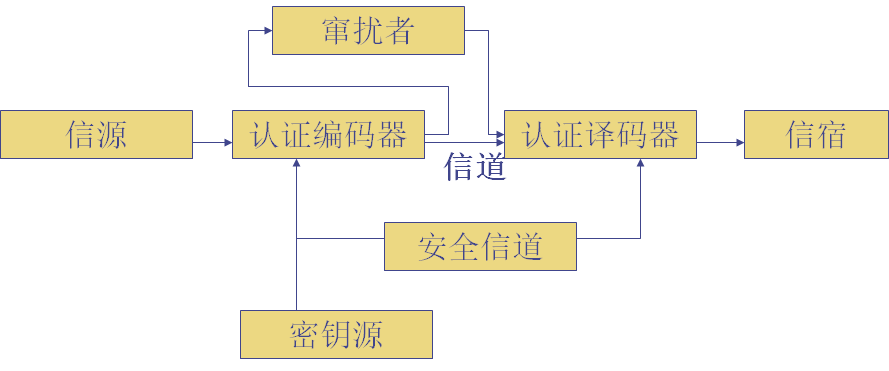• 消息加密
• 消息认证码MAC
• 需要密钥
• 哈希函数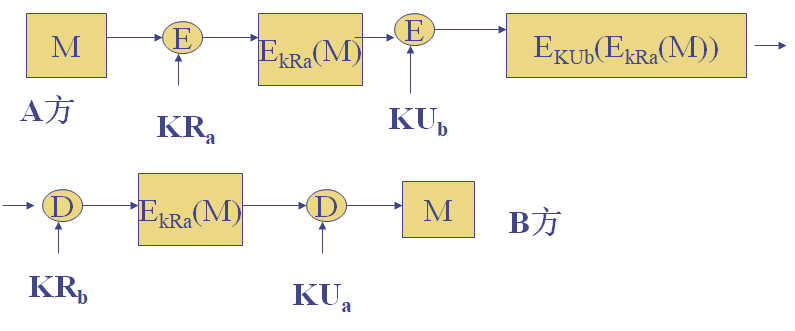## 消息认证码 MAC

MAC对给定消息，使用一个密钥，产生一个短小的定长数据分组。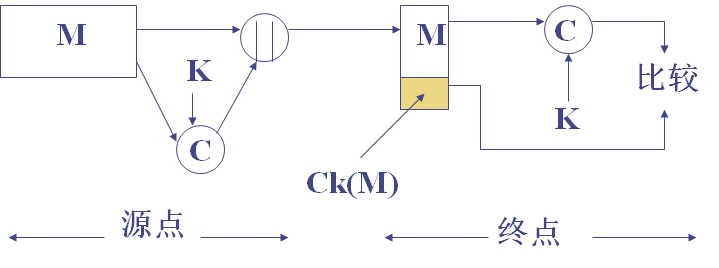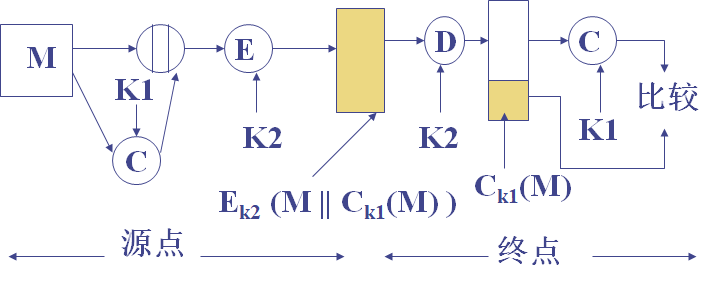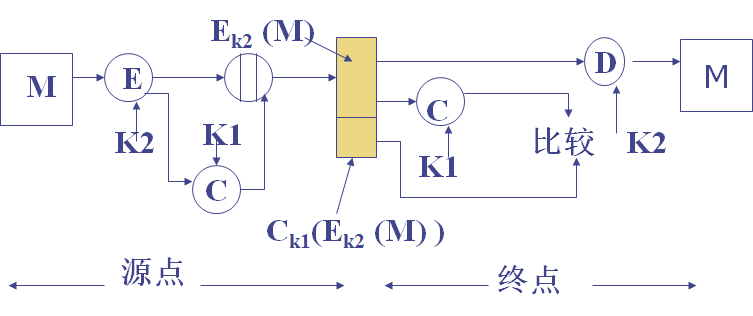• 适用于消息广播
• 认证的代价低
• 某些应用只关心消息的真实性
• 认证与保密的分离能提供结构上的灵活性
• 认证码可以延长消息的保护期限，同时能处理消息内容

• 将消息分为连续的64bit分组

\begin{align} C_1 &= E_K(M_1) \\ C_2 &= E_K(M_2\oplus C_1) \\ &\dots \\ C_n &= E_K(M_n\oplus C_{n-1}) \end{align}

## 哈希函数

• $$E_K(M||H(M))$$$• 提供保密与鉴别 • $$M||E_K(H(M))$$$
• 提供鉴别
• $$M||E_{K_{R_a}}(H(M))$$\$
• 提供鉴别与数字签名

### Merkle-Damgard结构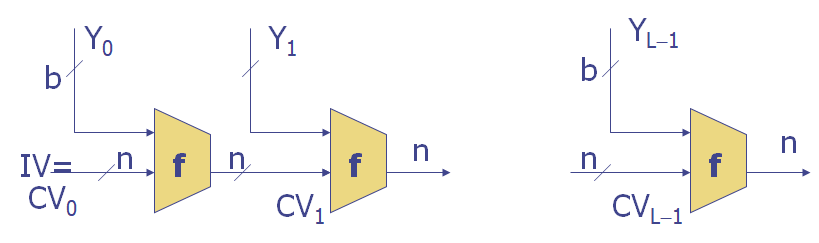$CV_i = f(CV_{i-1}, Y_{i-1}) \\ H(M) = CV_L$

## MD5

• 添加填充位，满足$$length\equiv448\bmod512$$
• 添加长度，用64bit表示，若超过只取低64位。
• 使用一个128bit缓存存放结果，表示为$$(A,B,C,D)$$
• 处理512bit的报文分组，核心是包含4个循环的压缩函数f，每个循环包括16步。
• 所有L个51bit的分组处理后，第L个阶段的输出作为128bit摘要输出。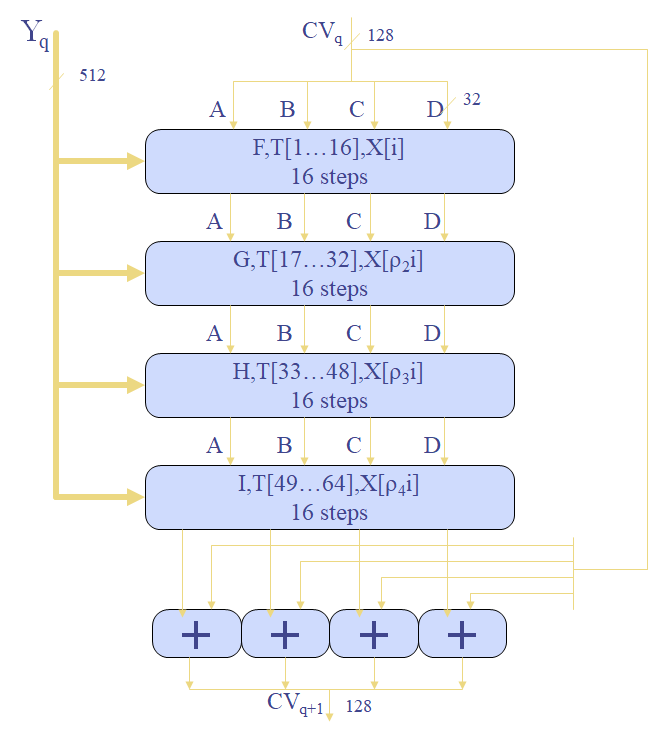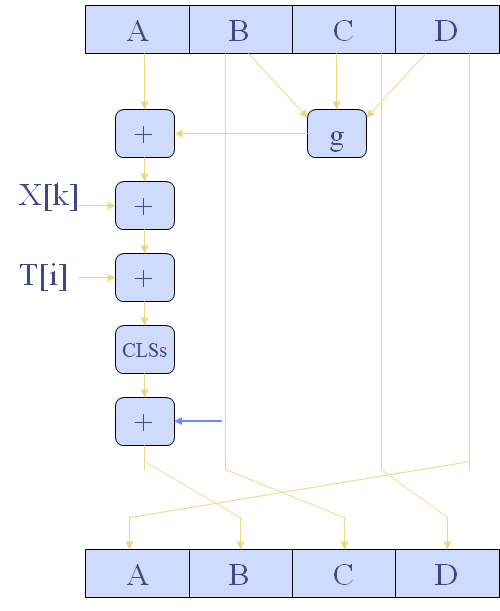MD4有3轮，每轮16步。

MD5每轮加上前一步的结果，有雪崩效应。

## SHA-1

SHA-1的函数有四轮，每轮20步。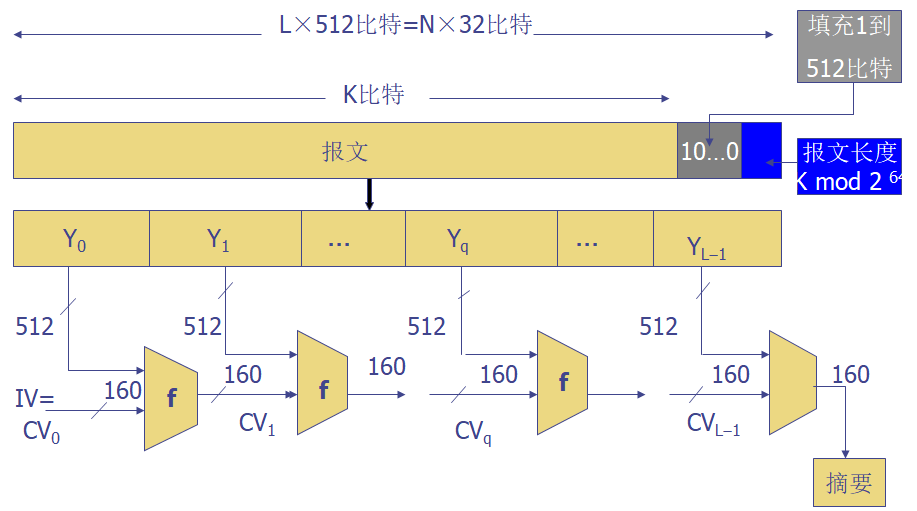## 安全性分析

• SHA = MD4 + 扩展变换 + 外加一轮 + 更好的雪崩
• MD5 = MD4 + 改进的比特杂凑 + 外加一轮 + 更好的雪崩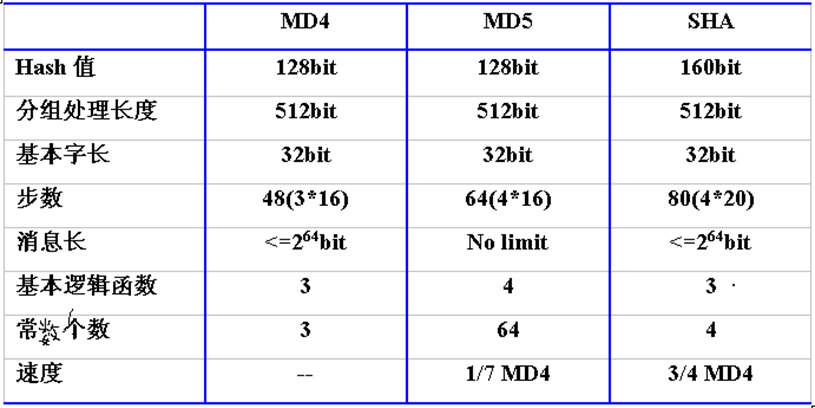k个人中，至少存在两人生日相同的概率为 $P(365, k) = 1 - \frac {365!} {(365-k)365^k}$